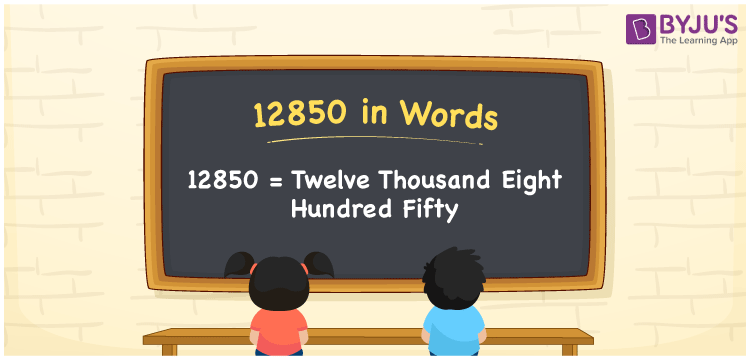# 12850 in Words

Twelve Thousand Eight Hundred Fifty is the number name for 12850. For instance, Arun pays a house rent of Rs. 12850 per month, then you can express, “Arun pays a house rent of Rupees Twelve Thousand Eight Hundred Fifty per month”. We know that 12850 is a cardinal number since it denotes a certain amount. Let us understand the method of converting the number 12850 in word form with simple tricks in this article.

 12850 in Words Twelve Thousand Eight Hundred Fifty Twelve Thousand Eight Hundred Fifty in numerical form 12850

## 12850 in English Words

Generally, we write numbers in words with the help of the letters of the English alphabet. Therefore, we can read the number 12850 in English as Twelve Thousand Eight Hundred Fifty.## How to Write 12850 in Words?

The number 12850 is a five-digit number. Hence, let us make a chart of 5 columns to depict the place value chart for 12850 as shown below.

 Ten Thousands Thousands Hundreds Tens Ones 1 2 8 5 0

Hence, we can write the expanded form as:

1 x Ten Thousand + 2 x Thousand + 8 x Hundred + 5 x Ten + 0 x One

= 1 x 10000 + 2 x 1000 + 8 x 100 + 5 x 10 + 0 x 1

= 10000 + 2000 + 800 + 50 + 0

= 10000 + 2000 + 800 + 50

= 12850

= Twelve Thousand Eight Hundred Fifty

Therefore, 12850 in words is written as Twelve Thousand Eight Hundred Fifty

Interesting way of writing 12850 in words

1 = One

12 = Twelve

128 = One Hundred and Twenty-Eight

1285 = One Thousand Two Hundred Eighty-Five

12850 = Twelve Thousand Eight Hundred Fifty

Thus, the word form of the number 12850 is Twelve Thousand Eight Hundred Fifty

12850 is a natural number that precedes 12851 and succeeds 12849

• 12850 in words – Twelve Thousand Eight Hundred Fifty
• Is 12850 an odd number? – No
• Is 12850 an even number? – Yes
• Is 12850 a perfect square number? – No
• Is 12850 a perfect cube number? – No
• Is 12850 a prime number? – No
• Is 12850 a composite number? – Yes

## Frequently Asked Questions on 12850 in Words

Q1

### Write 12850 in words.

12850 in words is written as Twelve Thousand Eight Hundred Fifty.
Q2

### Simplify 12000 + 850, and express in words.

Simplifying 12000 + 850, we get 12850. Thus, the number 12850 in words is Twelve Thousand Eight Hundred Fifty.
Q3

### 12850 is an even number. True or False.

True, the number 12850 is an even number.